# COMP7404 Computational Intelligence and Machine Learning

A Multi-agent Competitive Environment

• Other agents are planning against us
• Goals are in conflict (not necessarily)

Game Definition

• A game can be defined as
• s : States
• s0: Initial state
• Player(s) : Defines which player has the move
• Actions(s) : Returns a set of legal moves
• Result(s,a) : Defines the result of a move
• TerminalTest(s) : True when game is over, false otherwise
• Utility(s,p) : Defines the final numeric value for a game that ends in terminal state s for player p
• A game tree can be constructed
• Nodes are game states and edges are moves

Tic-Tac-Toe Game Tree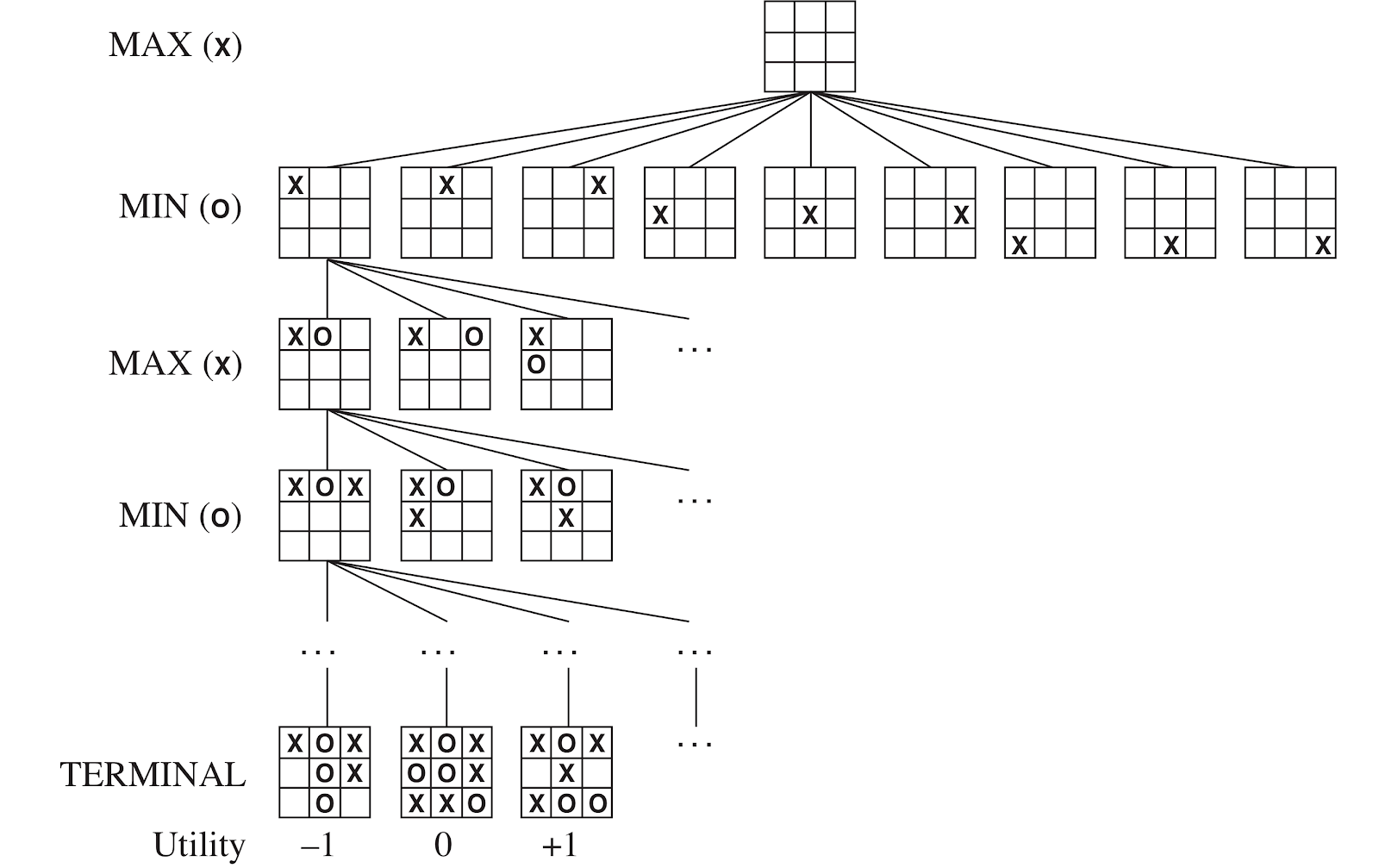Minimax Search

• A state-space search tree
• Players alternate turns
• Compute each node’s minimax value
• the best achievable utility against a rational (optimal) adversary
•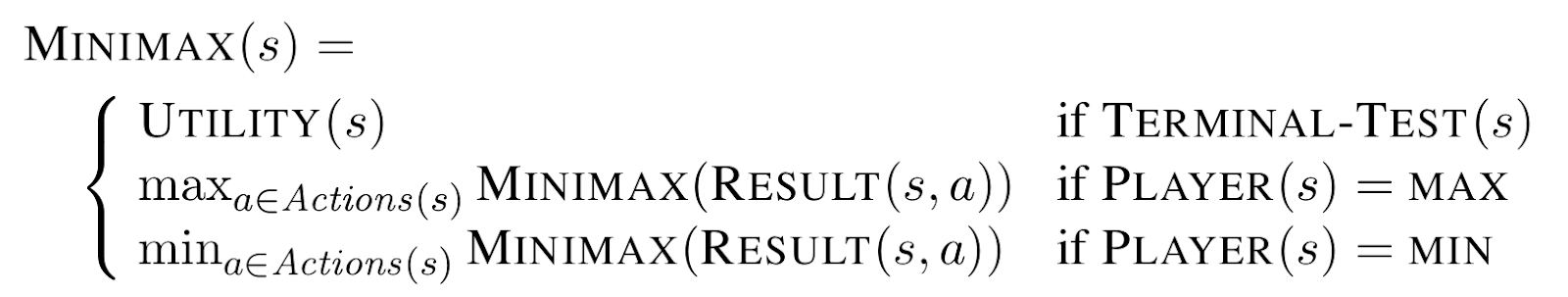• Will lead to optimal strategy
• Best achievable payoff against best play
• Example
•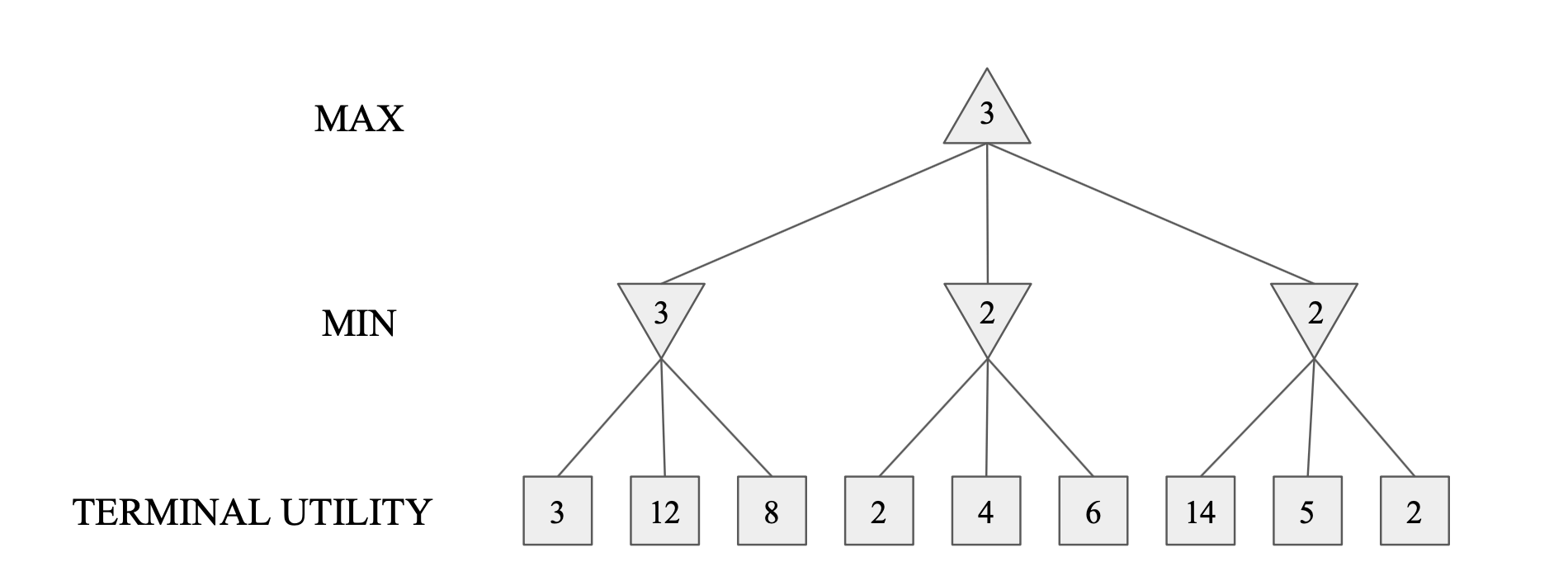• Implementation
•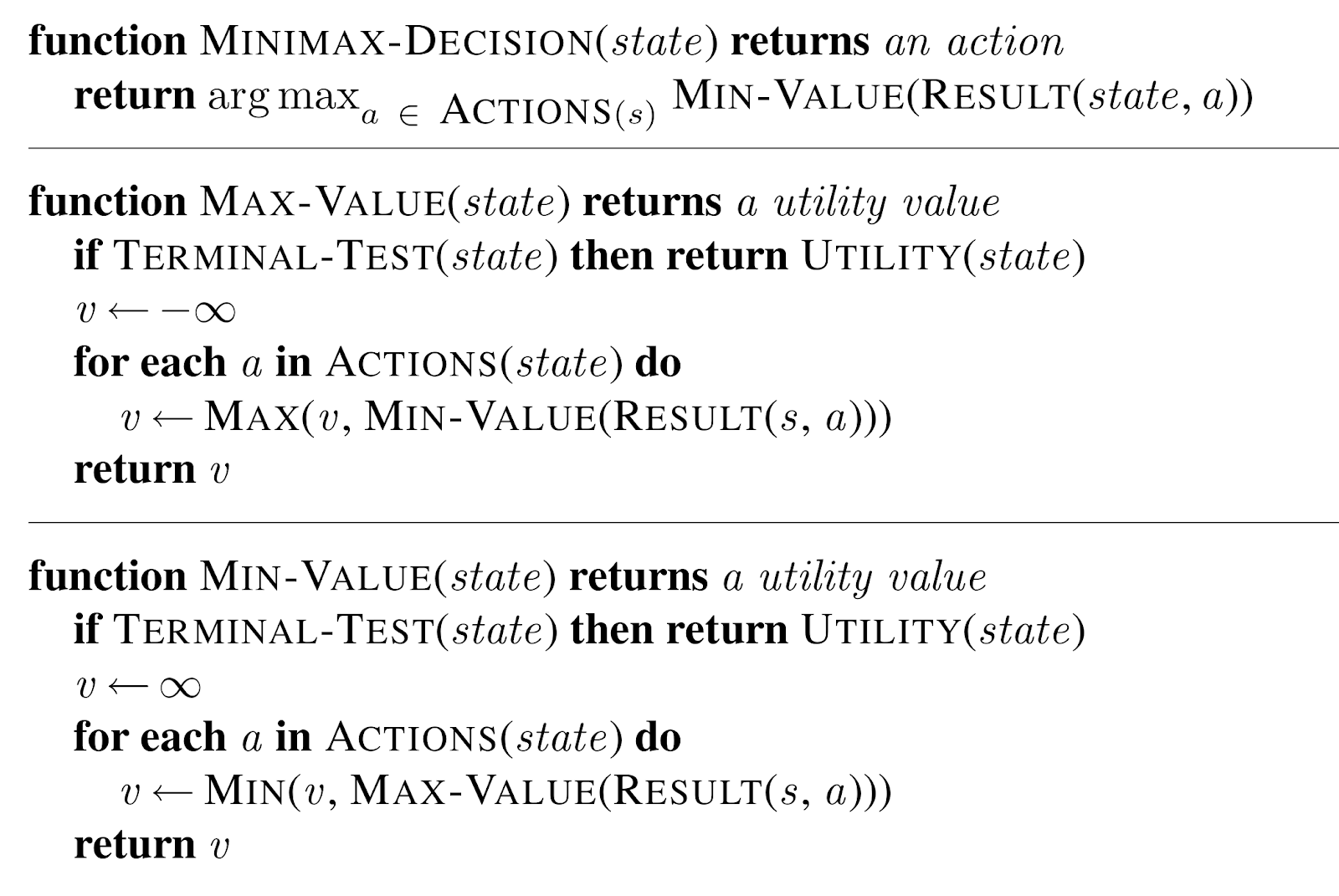• Properties
• Complete - Yes, if tree is finite
• Optimal - In general no, yes against an optimal opponent
• Time complexity - O(b^m)
• Space complexity - O(bm)

Depth-Limit Search (DLS)

• A depth limit search (DLS)
• Search only to a limited depth in the tree
• Replace terminal utilities with an evaluation function for non-terminal positions
• Problems
• Guarantee of optimal play is gone
• Need to design evaluation function
• An evaluation function
• An evaluation function Eval(s) scores non-terminals in depth-limited search
• An estimate of the expected utility of the game from a given position
• Ideal function
• The actual minimax value of the position
• The performance of a game-playing program depends strongly on the quality of its evaluation functio

𝛼-𝛽 Pruning Algorithm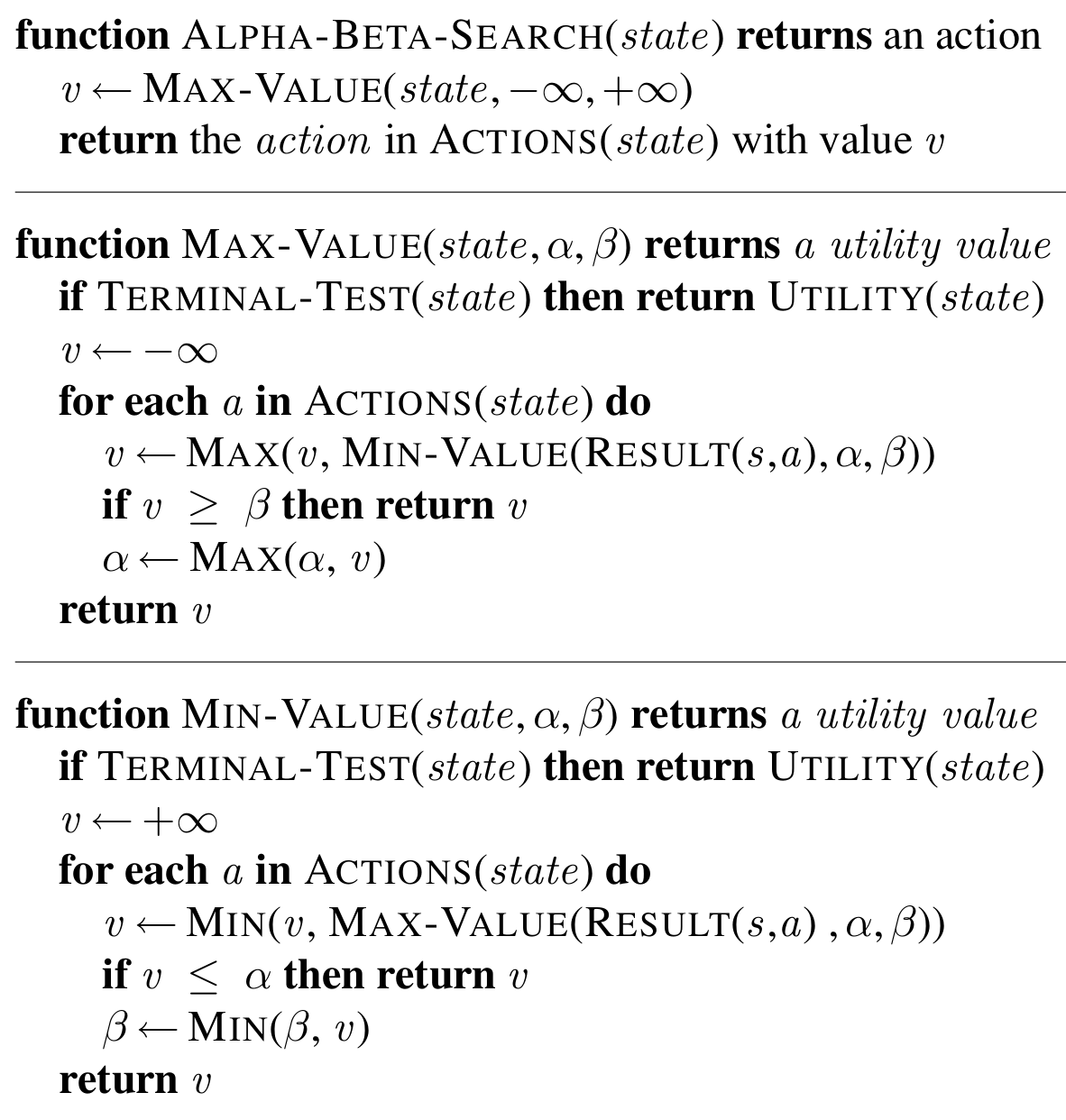• Min version
• Consider Min’s value at some node n
• n will decrease (or stay constant) while the descendants of n are examined
• Let m be the best value that Max can get at any choice point along the current path from the root
• If n becomes worse (<) than m
• Max will avoid it
• Stop considering n’s other children
• Max version is symmetric
• Properties
• Pruning has no effect on the minimax value at the root
• Values of intermediate nodes might be wrong
• Action selection not appropriate for this simple version of alpha-beta pruning
• Move ordering
• The effectiveness of alpha-beta pruning is highly dependent on the order in which states are examined
• It is worthwhile to try to examine first the successors that are likely best
• Examine only O(b^(m/2)) nodes to pick the best move, instead of O(bm) for minimax

A Reference Note

Expectimax Search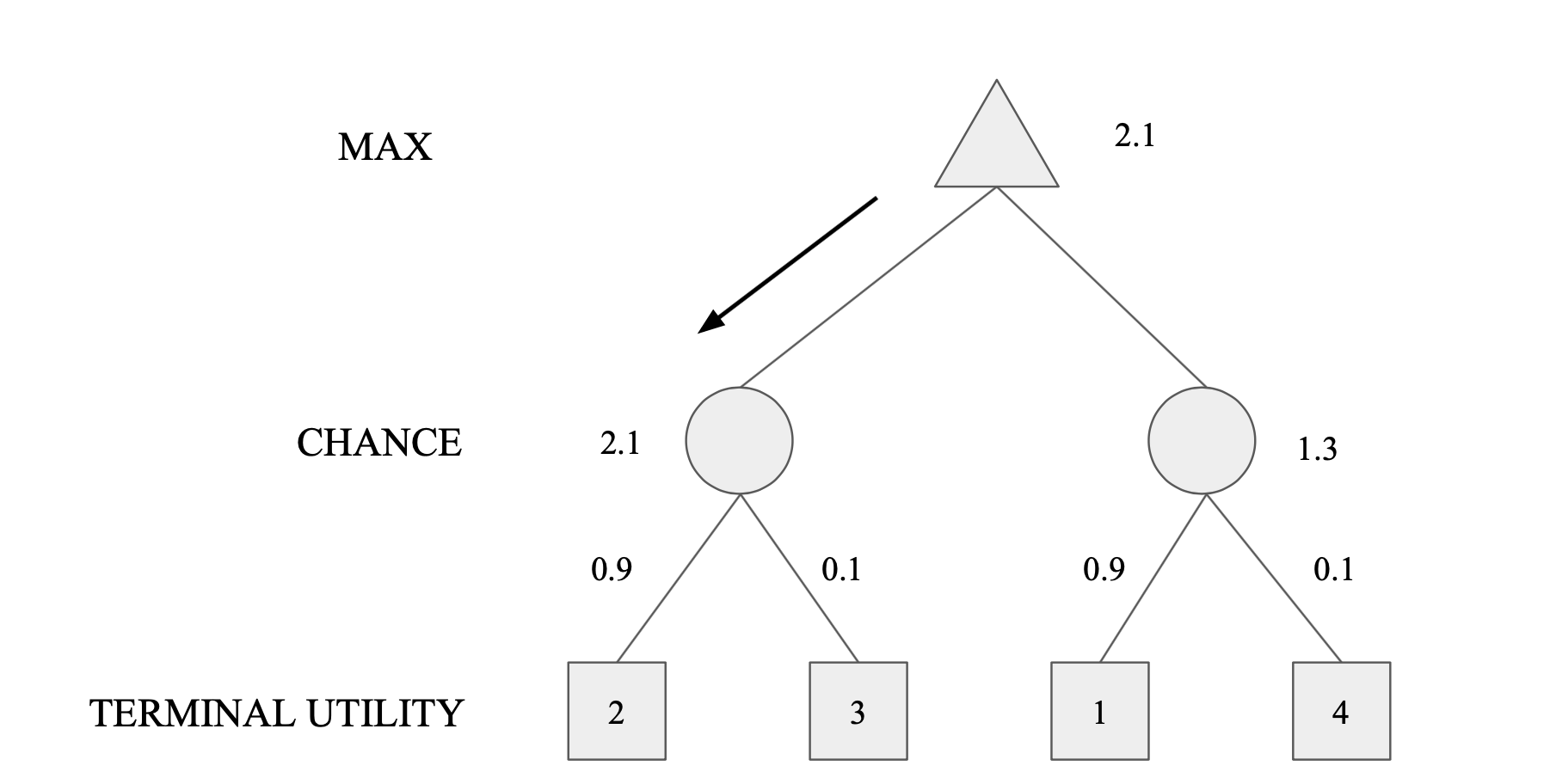• Values reflect average case outcomes, not worst case outcomes
• Expectimax search computes the expected score under optimal play
• Max nodes as in minimax search
• Chance nodes are like min nodes but the outcome is uncertain
• Calculate their expected utilities
• i.e., take weighted average of children
• Expectiminimax
• Environment is an extra “random agent” player that moves after each min/max agent

Multi-Agent Utilities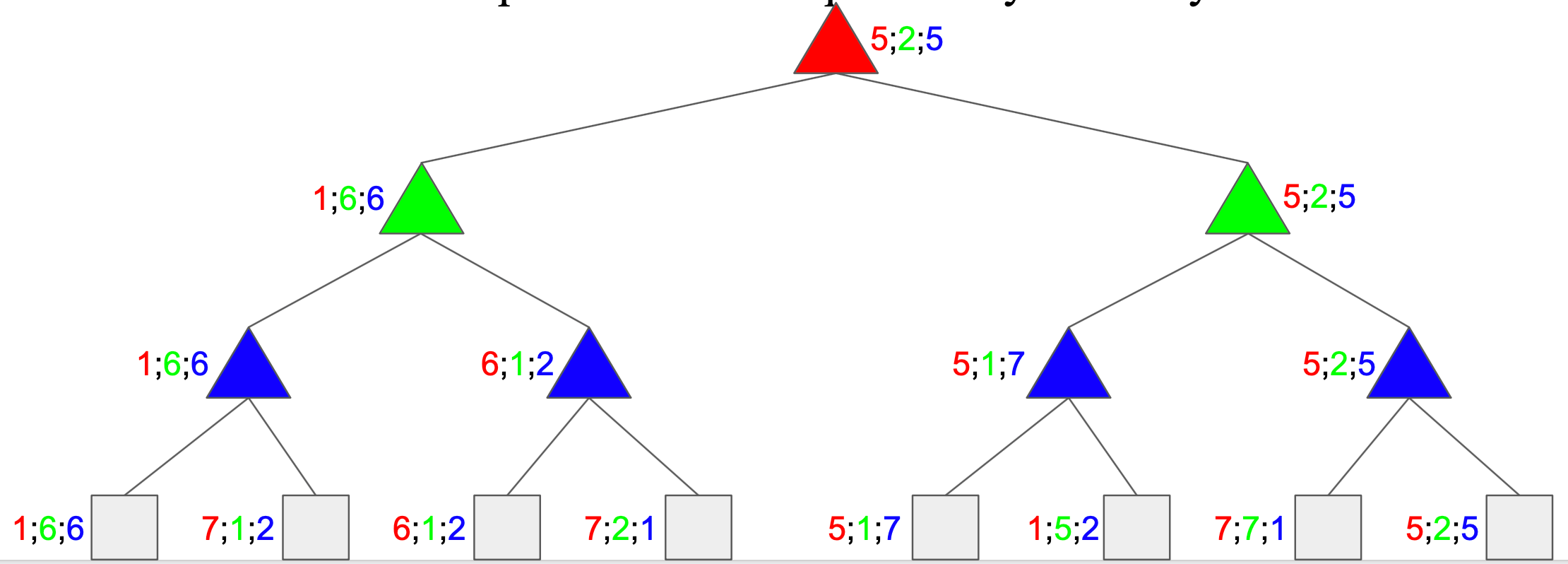• Generalisation of minimax
• Terminals and nodes have utility vectors
• Each player maximizes its own component
• Gives rise to cooperation and competition dynamically

## 相关文章

2020-11-11
xDeepFM for Recommender Systems - 推荐系统
2020-10-04
COMP7404 Topic 2 Beyond Classical Search
2020-09-16
COMP7404 Topic 1 Solving Problems by Searching

Yu Zhang